# Clone a Linked List with next and Random Pointer

• Difficulty Level : Hard
• Last Updated : 28 Jul, 2022

An example of linked list with a random pointerGiven a linked list of size N where each node has two links: one pointer points to the next node and the second pointer points to any node in the list. The task is to create a clone of this linked list in O(N) time

Note: The pointer pointing to the next node is ‘next‘ pointer and the one pointing to an arbitrary node is called ‘arbit’ pointer as it can point to any arbitrary node in the linked list.

An example of the linked list is shown in the below image: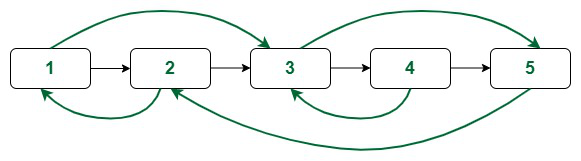An example of linked list with a random pointerAn example of linked list with a random pointerApproach 1 (Using Extra Space): The idea to solve this problem is:

First create a single linked list with only the ‘next’ pointer and use a mapping to map the new nodes to their corresponding nodes in the given linked list. Now use this mapping to point the arbitrary node from any node in the newly created list.

Follow the steps mentioned beloved to implement the above idea:

• Create a duplicate (say Y) for each node (say X) and map them with corresponding old nodes (say mp, So mp[X] = Y).
• Create the single linked list of the duplicate nodes where each node only has the ‘next’ pointer.
• Now iterate over the old linked list and do the following:
• Find the duplicate node mapped with the current one. (i.e., if the current node is X then duplicate is mp[x])
• Make the arbit pointer of the duplicate node pointing to the duplicate of the current->arbit node (i.e., mp[x]->arbit will point to mp[X->arbit]).
• The linked list created in this way is the required linked list.

Follow the illustration below for a better understanding:

Illustration:

Consider the linked list shown below:The green links are the arbit pointers

Creating copy of Nodes and next pointer:

Initially create single linked list of duplicate nodes with only the next pointers and map them with the old ones.
Here the blue coloured links are used to show the mapping.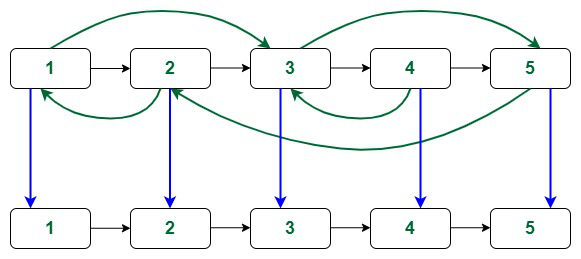New linked list mapped with old nodes

Now iterating the old array and update the arbit pointers as mentioned in the approach. The green coloured links are the arbit pointers.

At first node: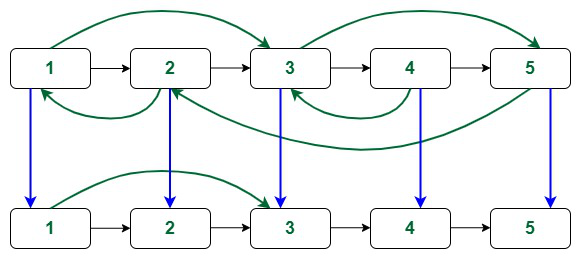Linking arbit pointer of duplicate of 1st node

At sconde node: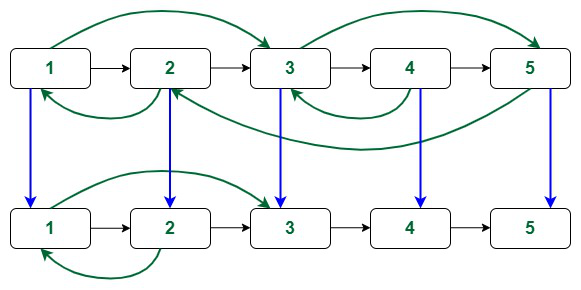Linking arbit pointer of duplicate of 2nd node

At third node: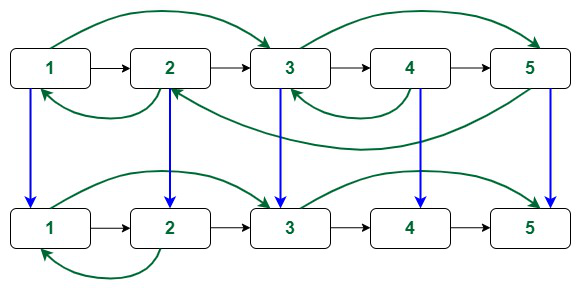Linking arbit pointer of duplicate of 3rd node

At fourth node: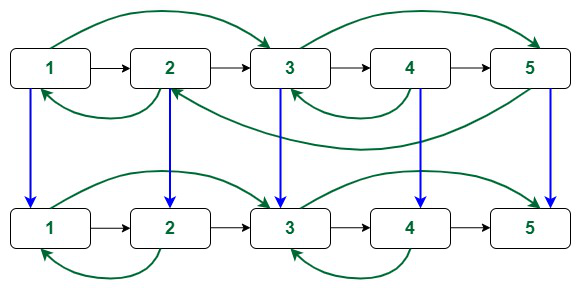Linking arbit pointer of duplicate of 4th node

At fifth node: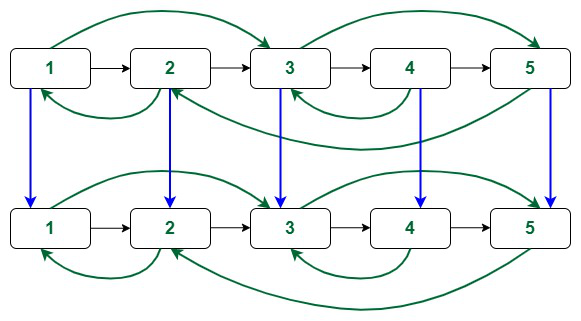Linking arbit pointer of duplicate of 5th node

The final linked list is as shown below: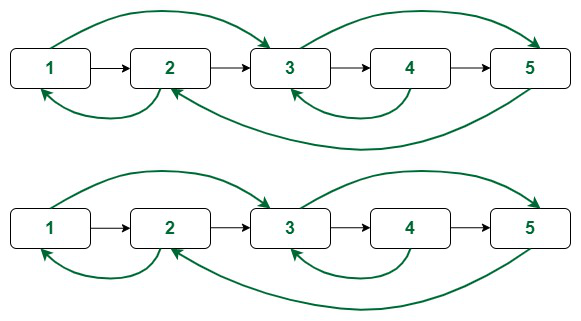The original and the clone

Below is the implementation of the above approach:

## C++14

 `// C++ code to implement the approach` `#include ``using` `namespace` `std;` `// Structure of a node of linked list``struct` `Node {``    ``int` `val;``    ``Node* next;``    ``Node* arbit;``  ` `    ``// Constructor``    ``Node(``int` `x)``    ``{``        ``this``->val = x;``        ``this``->next = NULL;``        ``this``->arbit = NULL;``    ``}``};` `// Function to clone the linked list``Node* cloneLinkedList(Node* head)``{``    ``// Map to store the mapping of``    ``// old nodes with new ones``    ``unordered_map mp;``    ``Node *temp, *nhead;``  ` `    ``// Duplicate of the first node``    ``temp = head;``    ``nhead = ``new` `Node(temp->val);``    ``mp[temp] = nhead;``  ` `    ``// Loop to create duplicates of nodes``    ``// with only next pointer``    ``while` `(temp->next != NULL) {``        ``nhead->next``            ``= ``new` `Node(temp->next->val);``        ``temp = temp->next;``        ``nhead = nhead->next;``        ``mp[temp] = nhead;``    ``}``    ``temp = head;``  ` `    ``// Loop to clone the arbit pointers``    ``while` `(temp != NULL) {``        ``mp[temp]->arbit = mp[temp->arbit];``        ``temp = temp->next;``    ``}``  ` `    ``// Return the head of the clone``    ``return` `mp[head];``}` `// Function to print the linked list``void` `printList(Node* head)``{``    ``cout << head->val << ``"("``         ``<< head->arbit->val << ``")"``;``    ``head = head->next;``    ``while` `(head != NULL) {``        ``cout << ``" -> "` `<< head->val << ``"("``             ``<< head->arbit->val << ``")"``;``        ``head = head->next;``    ``}``    ``cout << endl;``}` `// Driver code``int` `main()``{``    ``// Creating a linked list with random pointer``    ``Node* head = ``new` `Node(1);``    ``head->next = ``new` `Node(2);``    ``head->next->next = ``new` `Node(3);``    ``head->next->next->next = ``new` `Node(4);``    ``head->next->next->next->next``        ``= ``new` `Node(5);``    ``head->arbit = head->next->next;``    ``head->next->arbit = head;``    ``head->next->next->arbit``        ``= head->next->next->next->next;``    ``head->next->next->next->arbit``        ``= head->next->next;``    ``head->next->next->next->next->arbit``        ``= head->next;``  ` `    ``// Print the original list``    ``cout << ``"The original linked list:\n"``;``    ``printList(head);``  ` `    ``// Function call``    ``Node* sol = cloneLinkedList(head);``  ` `    ``cout << ``"The cloned linked list:\n"``;``    ``printList(sol);``  ` `    ``return` `0;``}`

Time Complexity: O(N)
Auxiliary Space: O(N)

Approach 2 (Using Constant Extra Space):  The idea to solve is using constant extra space is:

Create duplicate of a node and insert it in between that node and the node just next to it.

Now for a node X its duplicate will be X->next and the arbitrary pointer of the duplicate will point to X->arbit->next [as that is the duplicate of X->arbit]

Follow the steps mentioned below to implement the idea:

• Create the copy of node 1 and insert it between node 1 and node 2 in the original Linked List, create the copy of node 2 and insert it between 2nd and 3rd node and so on. Add the copy of N after the Nth node
• Now copy the arbitrary link in this fashion:

original->next->arbitrary = original->arbitrary->next

• Now restore the original and copy linked lists in this fashion in a single loop.

original->next = original->next->next;
copy->next = copy->next->next;

• Make sure that the last element of original->next is NULL.

Refer the below post for implementation of this method.
Clone a linked list with next and random pointer in O(1) space
Time Complexity: O(N)
Auxiliary Space: O(1)

Thanks to Saravanan Mani for providing this solution. This solution works using constant space.

Related article:
Clone a linked list with next and random pointer | Set 2

My Personal Notes arrow_drop_up